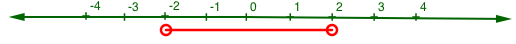### Home > AC > Chapter 10 > Lesson 10.3.1 > Problem10-107

10-107.

Solve the inequalities and equations below, if possible. Represent your solution on a number line.

1. $\left|x\right|+3<5$

Isolate the absolute value by subtracting $3$ from both sides.
$\left|x\right|+3<5$
$\left|x\right|+3-3<5-3$
$\left|x\right|<2$

Change the inequality into an equation and solve for $x$.
$\left|x\right|<2$
$\left|x\right|=2$
$x=2,-2$

Graph both values of $x$ on the number line, which divides the line into three regions.
Substitute a value from each region into the original inequality.
Whichever regions make the inequality true are the solutions.1. $5\left(2x+1\right)\ge30$

Change to an equation and solve for $x$.
$5\left(2x+1\right)\ge30$
$5\left(2x+1\right)=30$
$x=2.5$

Graph $x$ on a number line. Then check values from both regions to find the solution.

$x\ge2.5$
Don't forget to represent your solution with a number line!

1. $\frac{1}{x}-\frac{5}{2}=\frac{3}{2}$

Multiply both sides by $2x$ as Fraction Buster to eliminate the fractions.

$\left(\frac{1}{x}\right)2x-\left(\frac{5}{2}\right)2x=\left(\frac{3}{2}\right)2x$

$2-5x=3x$

$\textit{x}=\frac{1}{4}$

1. $-5-x>3-x$

Add $x$ to both sides.
$-5-x+x>3-x+x$
$-5>3$
Is this statement true? If so, then $x$ can be any real number. If not, then there is no real solution.

1. $3\sqrt{4-x}+1=13$

Subtract $1$ from both sides.Then divide by three. This will isolate the square root. Look inside to determine the solution.

1. $\left|x+1\right|\le4$

See the help for part (a).